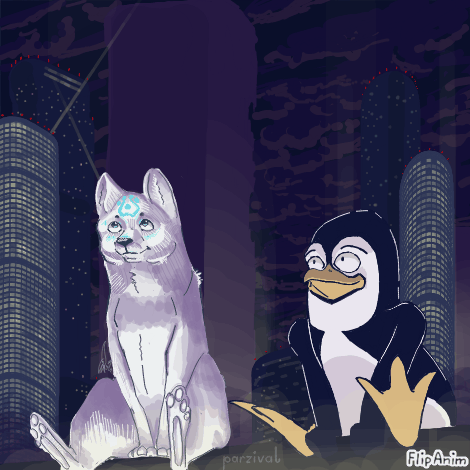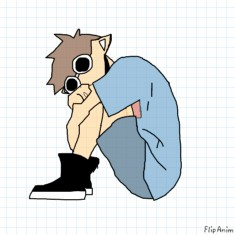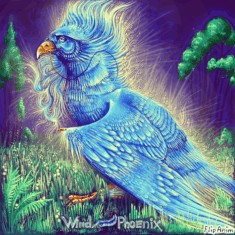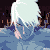- create flipbook animations online!
Starty sky :) (collab)31.10.202031.10.2020 17:03
wait your the one who made THIS this was my favorite anim since i ever started31.10.2020 17:06
:000000000000000000 :00000000000000 :)))))))))))))))))))))))))))))))))))))))))))))))))))))))))))))))))))))))))))))))))))))))))))))))))))))))))))))))))))))))))31.10.2020 17:11
:3 tysm! But check out Parzival, that FArtist and flipanimator in one time has a lot of great stuff! Also Parzival had drawn the background!31.10.2020 17:39
I'm really glad that you did the collab with me! I think they both work good together in this piece! Also, are you now mostly active on FlipAnim?31.10.2020 18:49
You could also try a collab with CatLady15. I bet your artstyles would complement each other greatly!!